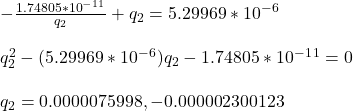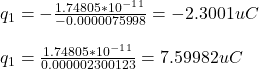## when their center-to-center separation is 50 cm. The spheres are then connected by a thin conducting wire. When the wire is removed, the sph

Question

when their center-to-center separation is 50 cm. The spheres are then connected by a thin conducting wire. When the wire is removed, the spheres repel each other with an electrostatic force of 0.2525 N. What were the initial charges on the spheres

in progress 0
3 weeks 2021-08-23T23:35:07+00:00 1 Answers 0 views 0

q1 = 7.6uC , -2.3 uC

q2 = 7.6uC , -2.3 uC

( q1 , q2 ) = ( 7.6 uC , -2.3 uC ) OR ( -2.3 uC , 7.6 uC )

Explanation:

Solution:-

– We have two stationary identical conducting spheres with initial charges ( q1 and q2 ). Such that the force of attraction between them was F = 0.6286 N.

– To model the electrostatic force ( F ) between two stationary charged objects we can apply the Coulomb’s Law, which states: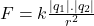Where,

k: The coulomb’s constant = 8.99*10^9

– Coulomb’s law assume the objects as point charges with separation or ( r ) from center to center.

– We can apply the assumption and approximate the spheres as point charges under the basis that charge is uniformly distributed over and inside the sphere.

– Therefore, the force of attraction between the spheres would be: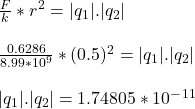Eq 1

Once, we connect the two spheres with a conducting wire the charges redistribute themselves until the charges on both sphere are equal ( q’ ). This is the point when the re-distribution is complete ( current stops in the wire).

– We will apply the principle of conservation of charges. As charge is neither destroyed nor created. Therefore,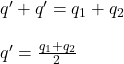– Once the conducting wire is connected. The spheres at the same distance of ( r = 0.5m) repel one another. We will again apply the Coulombs Law as follows for the force of repulsion (F = 0.2525 N ) as follows: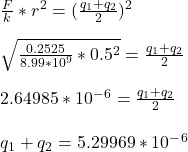.. Eq2

We have two equations with two unknowns. We can solve them simultaneously to solve for initial charges ( q1 and q2 ) as follows: